# Motion What Is Motion Motion is when an

• Slides: 47MotionWhat Is Motion? Motion is when an object changes place or position. To properly describe motion, you need to use the following: • • Start and end position? Movement relative to what? How far did it go? In what direction did it go?What Is Speed? Speed is the distance an object travels in a certain amount of time. To calculate speed, you use the following formula: Speed (s) = Distance (d) Time (t)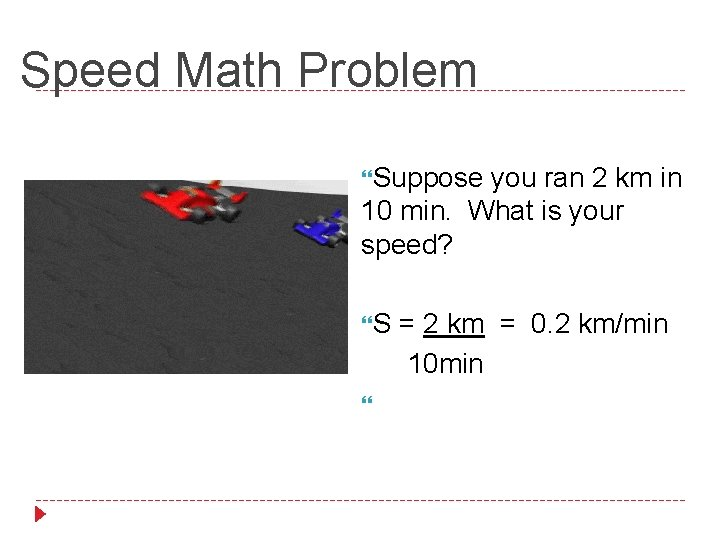Speed Math Problem Suppose you ran 2 km in 10 min. What is your speed? S = 2 km = 0. 2 km/min 10 minSpeed Practice Time!!!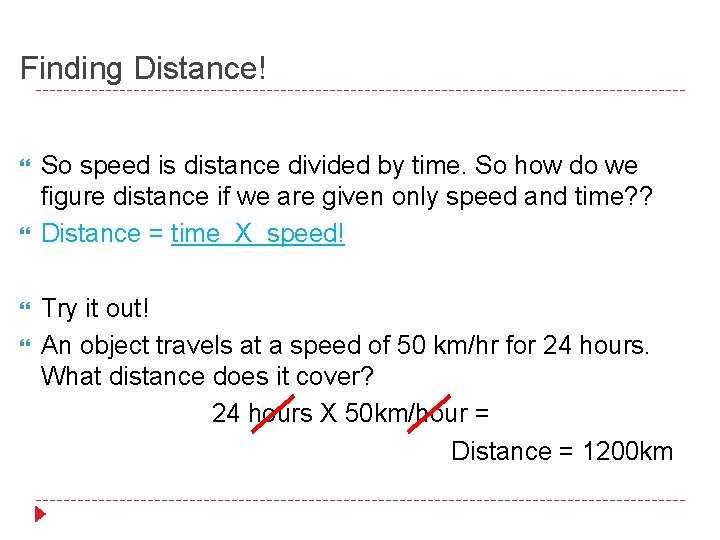Finding Distance! So speed is distance divided by time. So how do we figure distance if we are given only speed and time? ? Distance = time X speed! Try it out! An object travels at a speed of 50 km/hr for 24 hours. What distance does it cover? 24 hours X 50 km/hour = Distance = 1200 km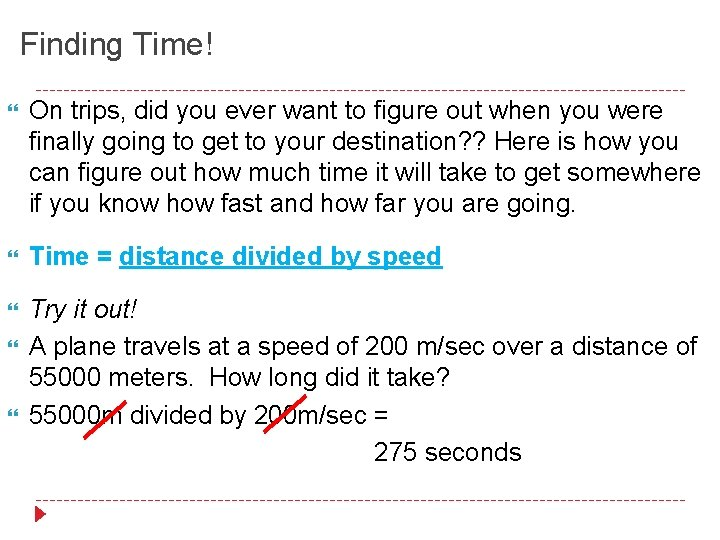Finding Time! On trips, did you ever want to figure out when you were finally going to get to your destination? ? Here is how you can figure out how much time it will take to get somewhere if you know how fast and how far you are going. Time = distance divided by speed Try it out! A plane travels at a speed of 200 m/sec over a distance of 55000 meters. How long did it take? 55000 m divided by 200 m/sec = 275 secondsTime and Distance PracticeRead Chapter 7 in your Physical Science book and answer the questions that I have handed out to you.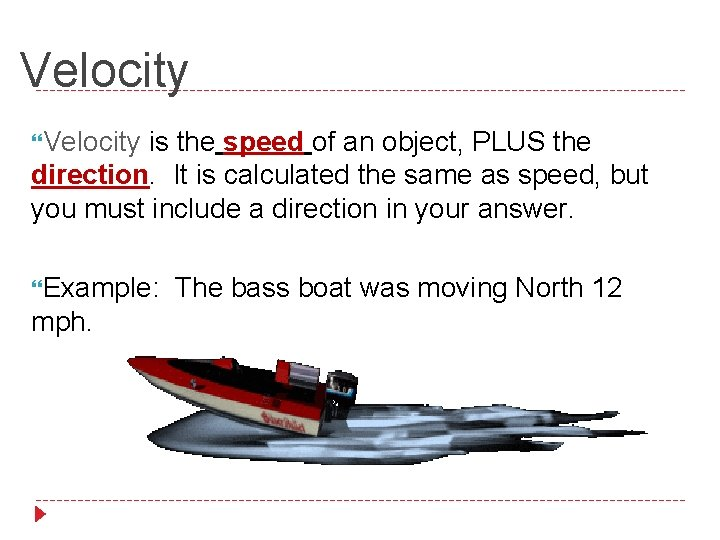Velocity is the speed of an object, PLUS the direction. It is calculated the same as speed, but you must include a direction in your answer. Example: The bass boat was moving North 12 mph.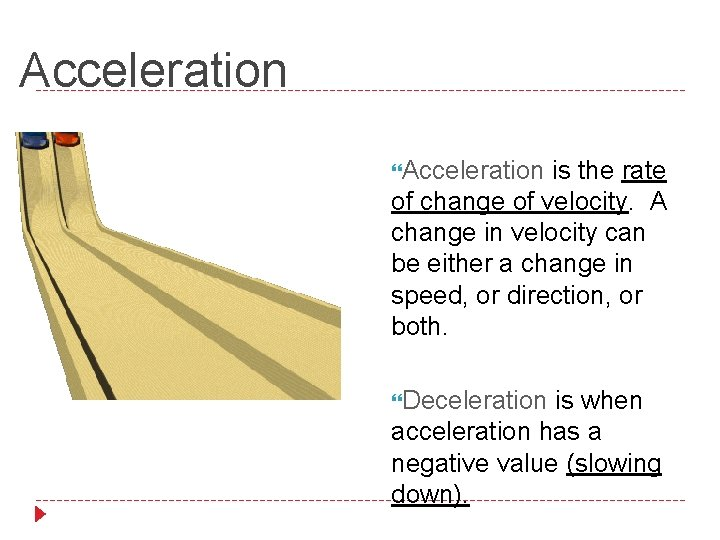Acceleration is the rate of change of velocity. A change in velocity can be either a change in speed, or direction, or both. Deceleration is when acceleration has a negative value (slowing down).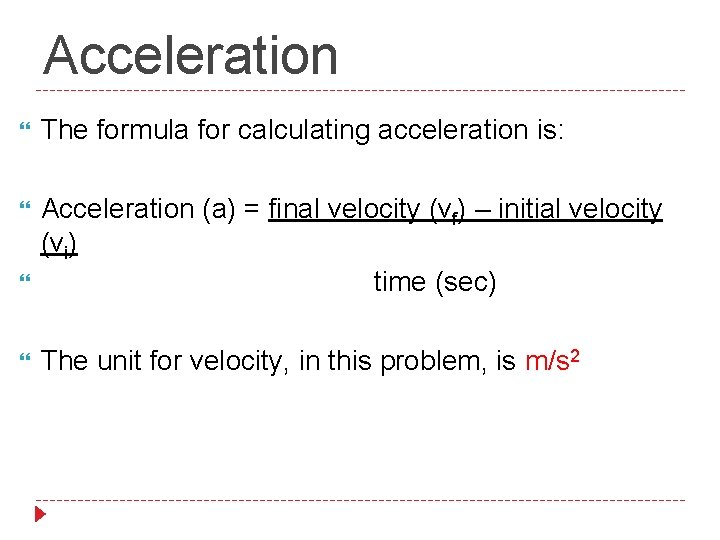Acceleration The formula for calculating acceleration is: Acceleration (a) = final velocity (vf) – initial velocity (vi) time (sec) The unit for velocity, in this problem, is m/s 2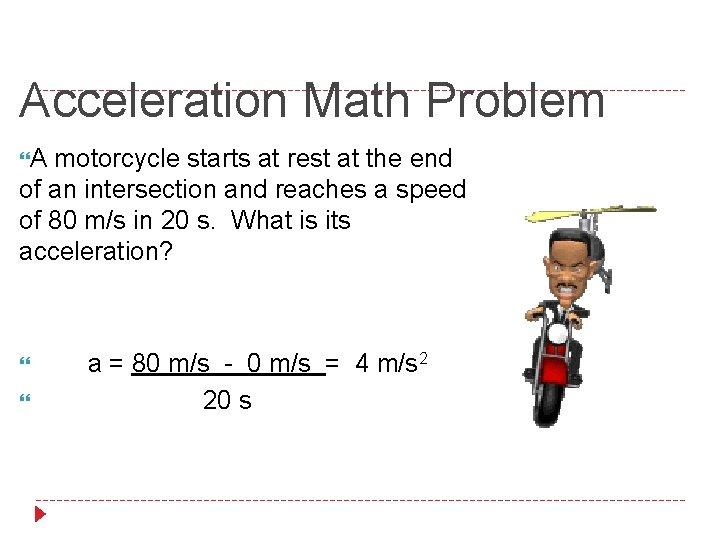Acceleration Math Problem A motorcycle starts at rest at the end of an intersection and reaches a speed of 80 m/s in 20 s. What is its acceleration? a = 80 m/s - 0 m/s = 4 m/s 2 20 sAcceleration Math Problem A skateboarder is moving in a straight line at a speed of 3 m/s and comes to a stop in 2 sec. What is his acceleration? a = 0 m/s - 3 m/s = -1. 5 m/s 2 2 m/s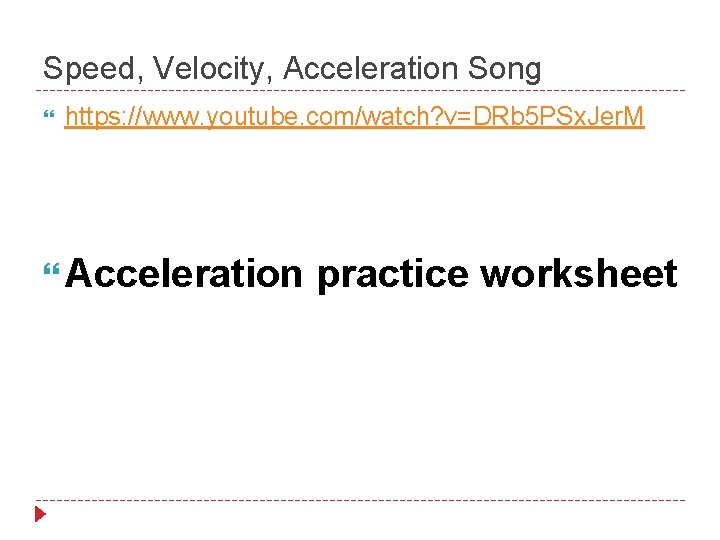Speed, Velocity, Acceleration Song https: //www. youtube. com/watch? v=DRb 5 PSx. Jer. M Acceleration practice worksheet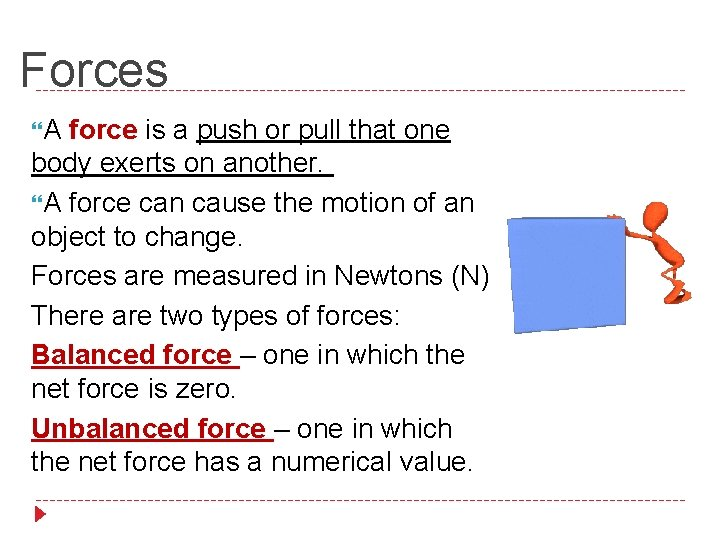Forces A force is a push or pull that one body exerts on another. A force can cause the motion of an object to change. Forces are measured in Newtons (N) There are two types of forces: Balanced force – one in which the net force is zero. Unbalanced force – one in which the net force has a numerical value.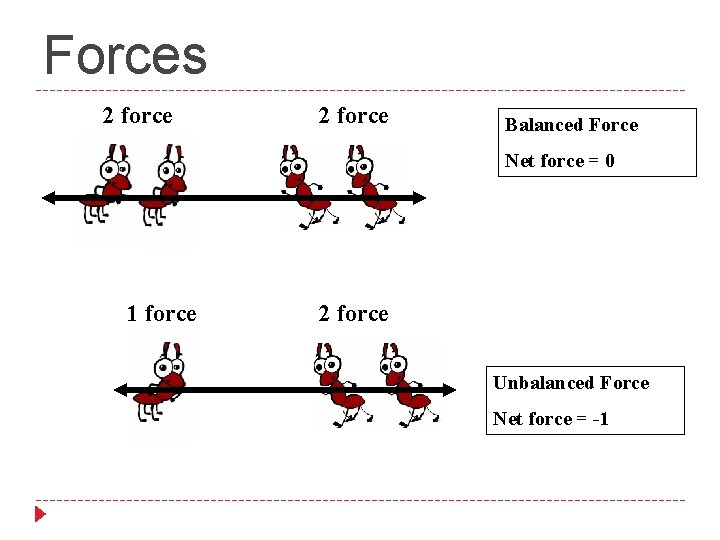Forces 2 force Balanced Force Net force = 0 1 force 2 force Unbalanced Force Net force = -1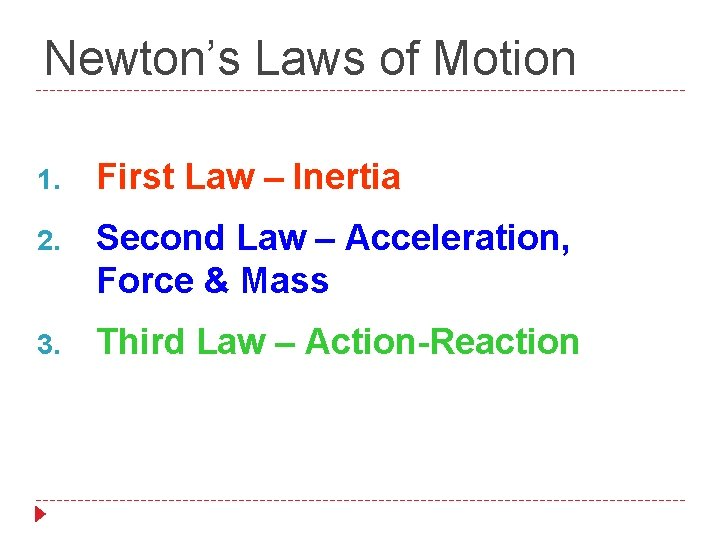Newton’s Laws of Motion 1. First Law – Inertia 2. Second Law – Acceleration, Force & Mass 3. Third Law – Action-Reaction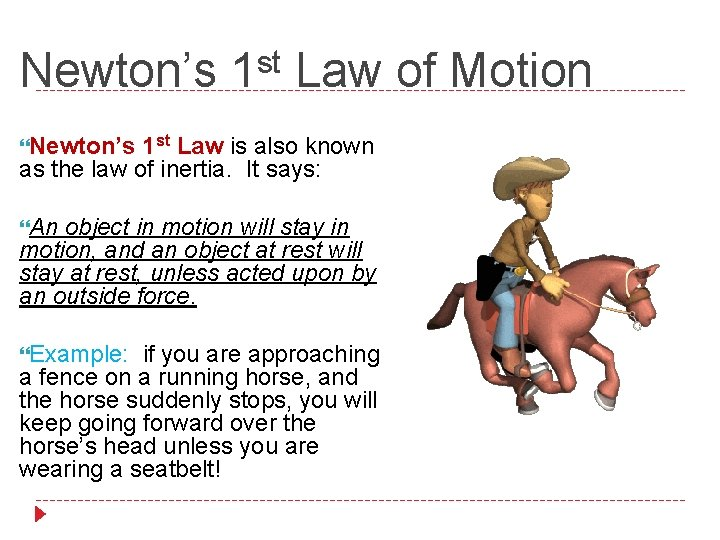Newton’s 1 st Law of Motion Newton’s 1 st Law is also known as the law of inertia. It says: An object in motion will stay in motion, and an object at rest will stay at rest, unless acted upon by an outside force. Example: if you are approaching a fence on a running horse, and the horse suddenly stops, you will keep going forward over the horse’s head unless you are wearing a seatbelt!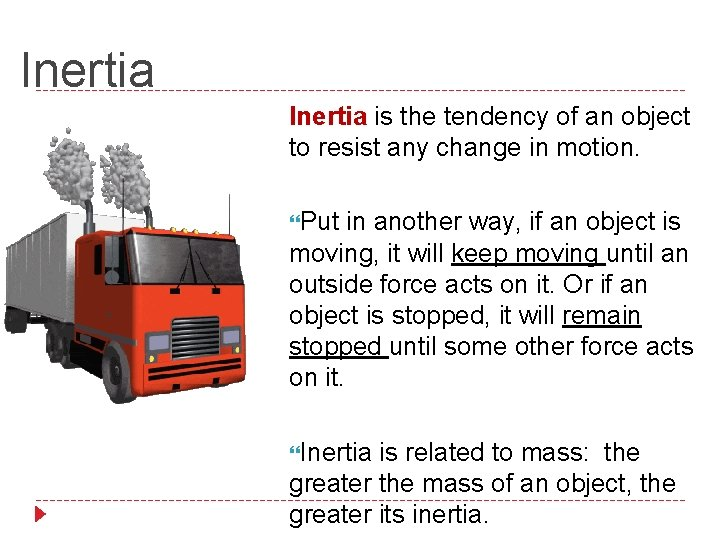Inertia is the tendency of an object to resist any change in motion. Put in another way, if an object is moving, it will keep moving until an outside force acts on it. Or if an object is stopped, it will remain stopped until some other force acts on it. Inertia is related to mass: the greater the mass of an object, the greater its inertia.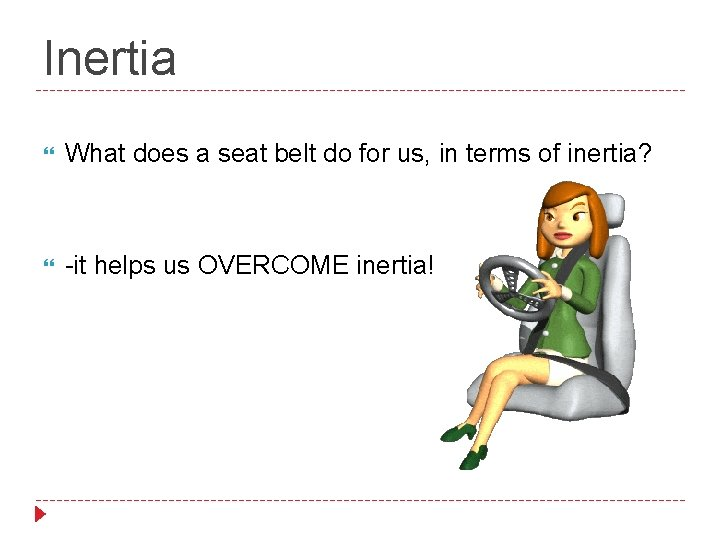Inertia What does a seat belt do for us, in terms of inertia? -it helps us OVERCOME inertia!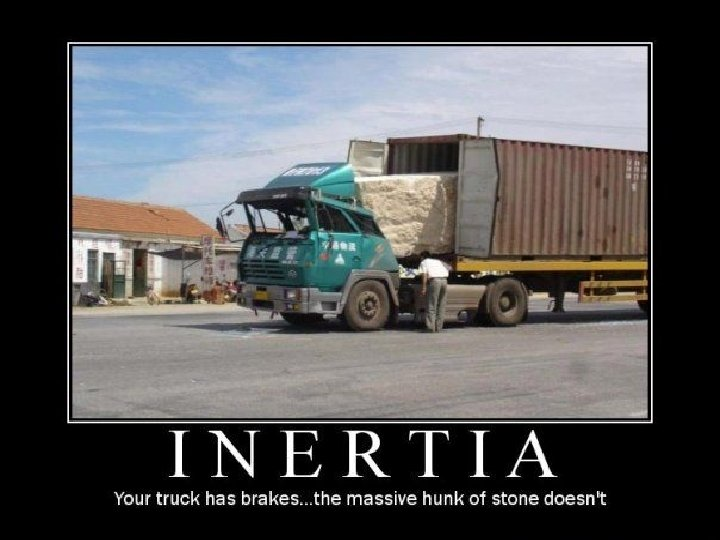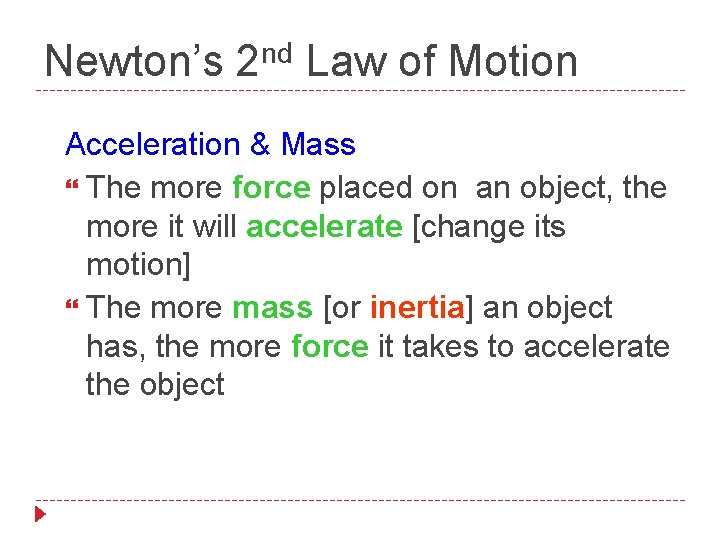nd Newton’s 2 Law of Motion Acceleration & Mass The more force placed on an object, the more it will accelerate [change its motion] The more mass [or inertia] an object has, the more force it takes to accelerate the objectrd Newton’s 3 Law Action – Reaction Forces are always produced in pairs with opposite directions & equal strengths For every force there is an equal and opposite force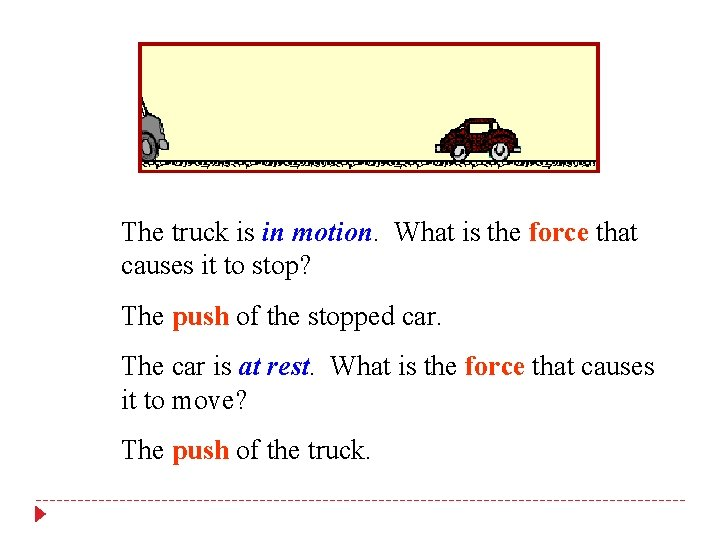The truck is in motion. What is the force that causes it to stop? The push of the stopped car. The car is at rest. What is the force that causes it to move? The push of the truck.What about the ladder on top of the truck? The ladder is in motion because the truck is in motion. When the truck stops, the ladder stays in motion. The truck is stopped by the force of the car, but the ladder is not. What force stops the ladder? Gravity.Why does the car move when it is hit by the truck? The heavy and moving truck has more force than the small, at rest car. Why does the truck stop moving when it hits the car? The force of the car pushing back on the truck, plus the force of friction between the massive truck and the road slow down, the stop the truck.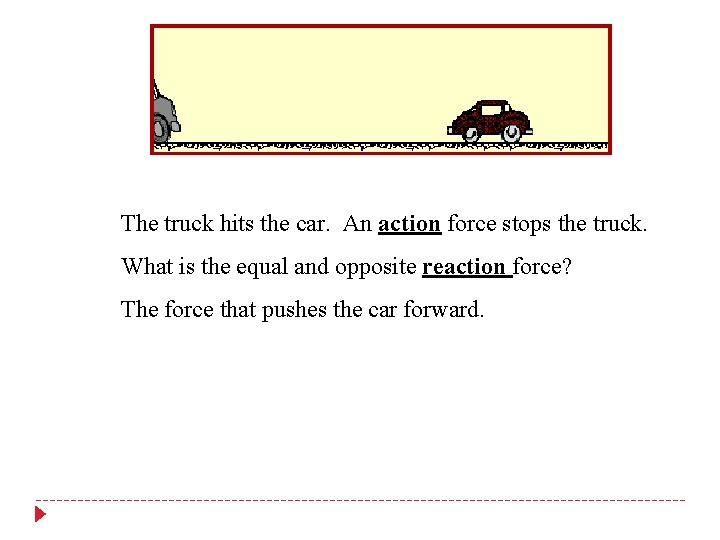The truck hits the car. An action force stops the truck. What is the equal and opposite reaction force? The force that pushes the car forward.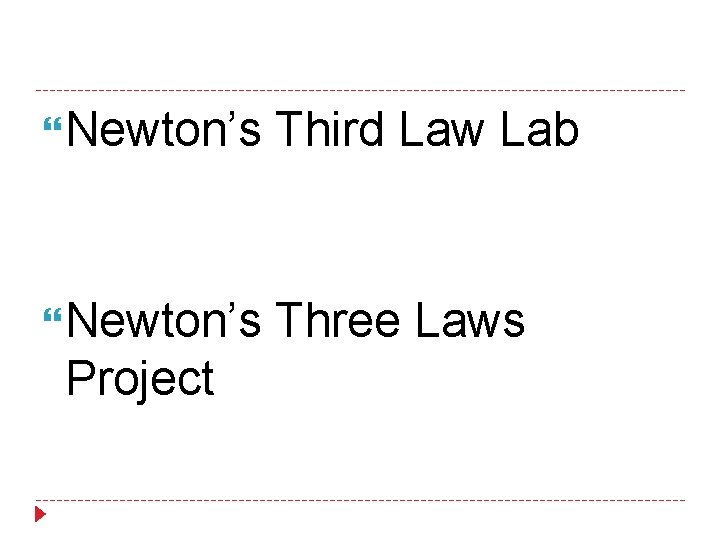Newton’s Third Law Lab Newton’s Three Laws Project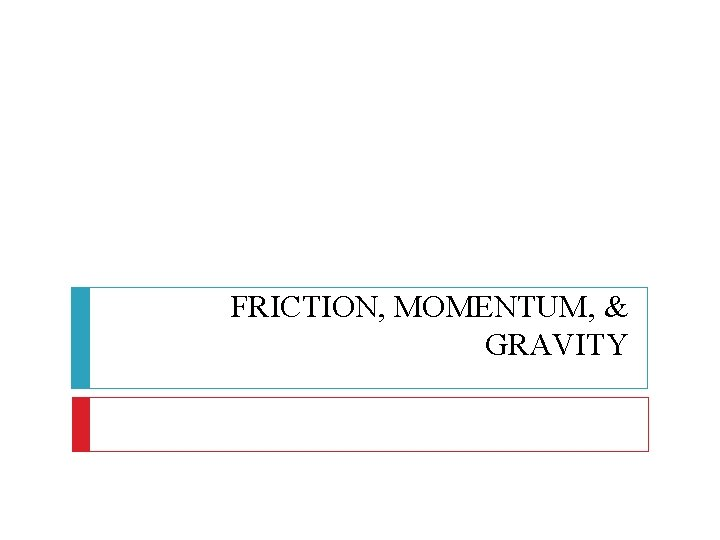FRICTION, MOMENTUM, & GRAVITY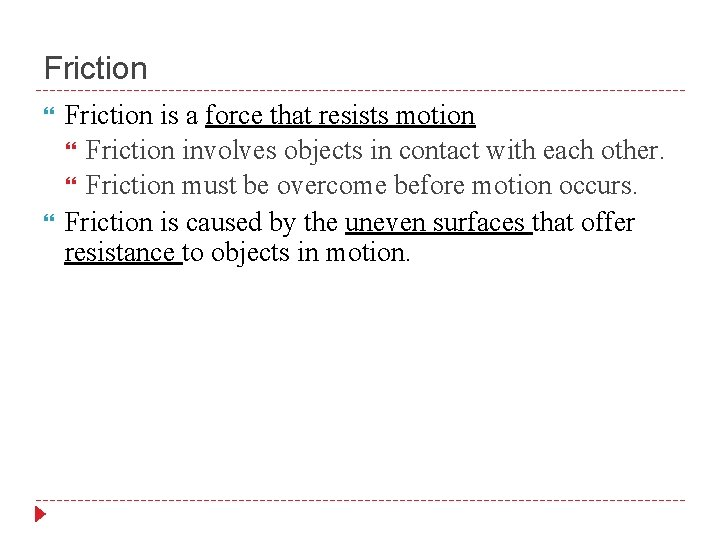Friction is a force that resists motion Friction involves objects in contact with each other. Friction must be overcome before motion occurs. Friction is caused by the uneven surfaces that offer resistance to objects in motion.Friction and Anti-Lock Brakes Attempts to make the tire exert a force larger than the material can handle causes the tire to “burn rubber” and actually reduces the force that the car is able to use. Antilock brakes sense the wheel rotation and “ease off” if it is close to stopping, maintaining static friction with the road and allowing better control of steering than if the wheels were locked.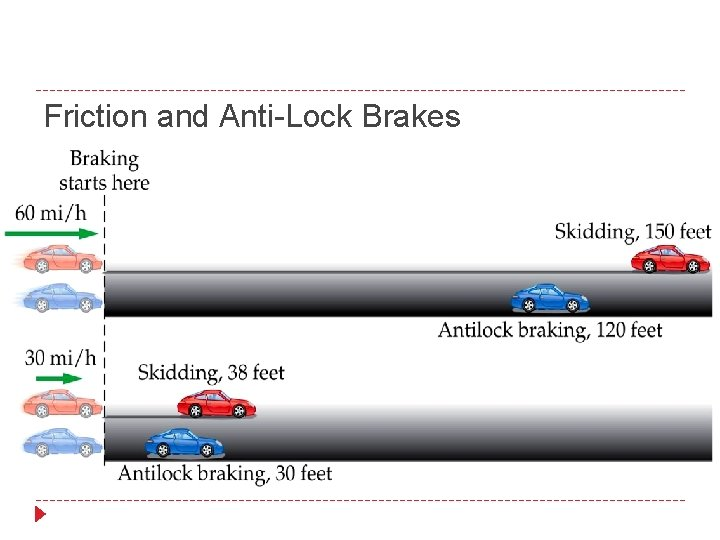Friction and Anti-Lock Brakes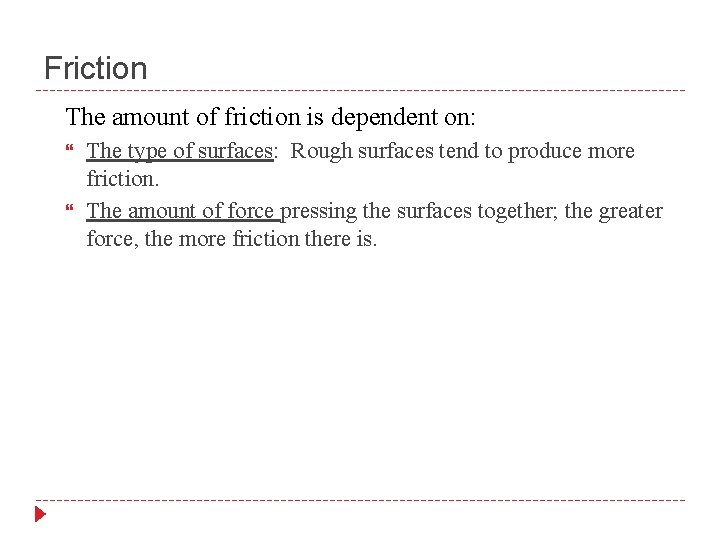Friction The amount of friction is dependent on: The type of surfaces: Rough surfaces tend to produce more friction. The amount of force pressing the surfaces together; the greater force, the more friction there is.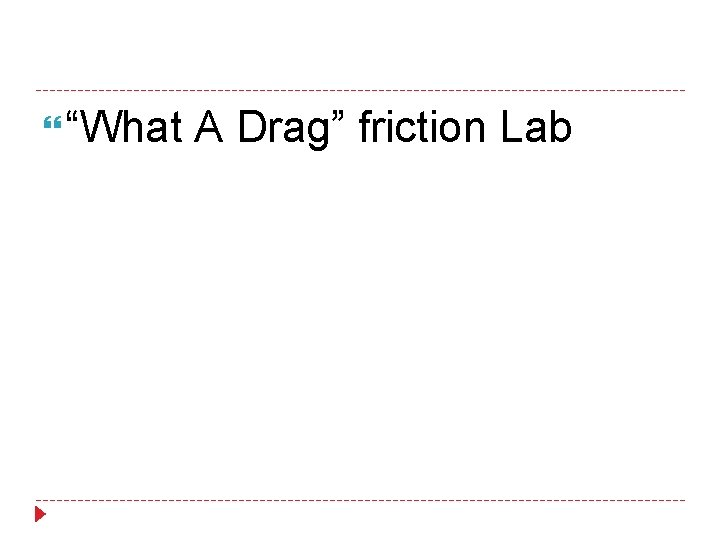“What A Drag” friction Lab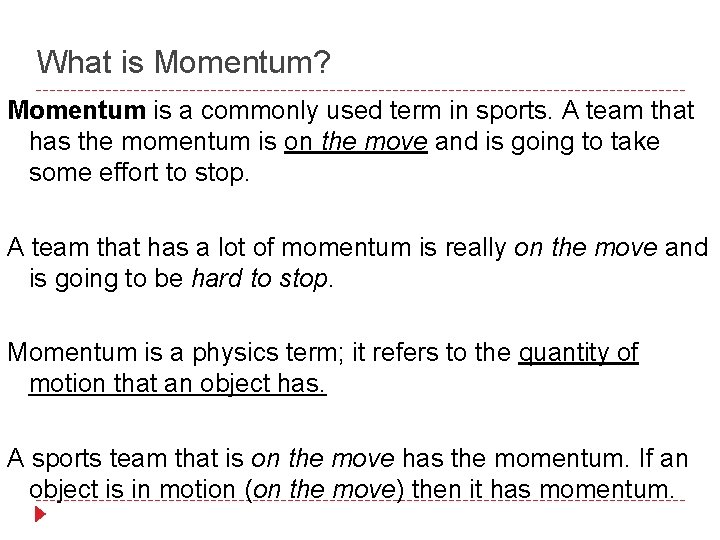What is Momentum? Momentum is a commonly used term in sports. A team that has the momentum is on the move and is going to take some effort to stop. A team that has a lot of momentum is really on the move and is going to be hard to stop. Momentum is a physics term; it refers to the quantity of motion that an object has. A sports team that is on the move has the momentum. If an object is in motion (on the move) then it has momentum.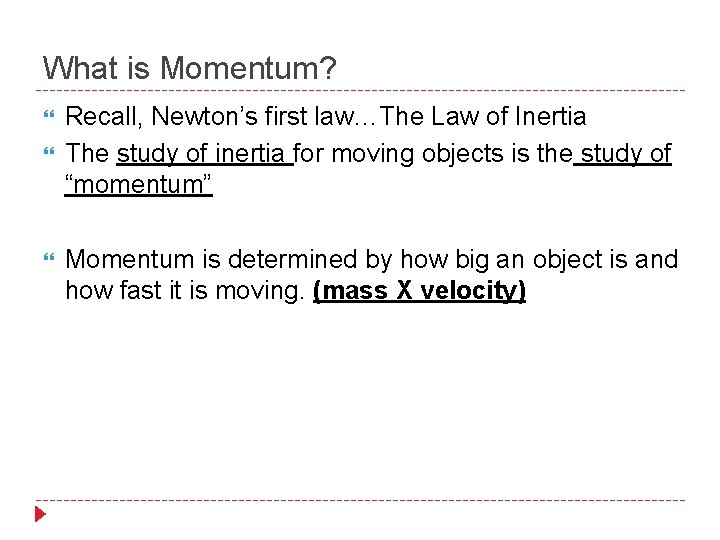What is Momentum? Recall, Newton’s first law…The Law of Inertia The study of inertia for moving objects is the study of “momentum” Momentum is determined by how big an object is and how fast it is moving. (mass X velocity)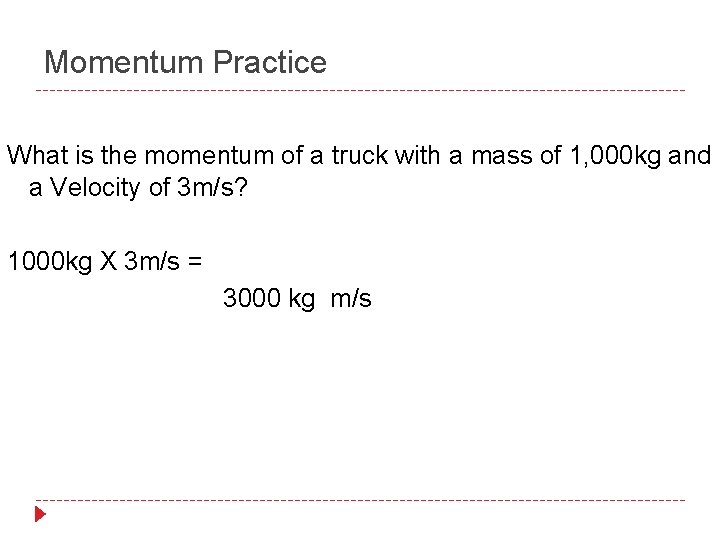Momentum Practice What is the momentum of a truck with a mass of 1, 000 kg and a Velocity of 3 m/s? 1000 kg X 3 m/s = 3000 kg m/s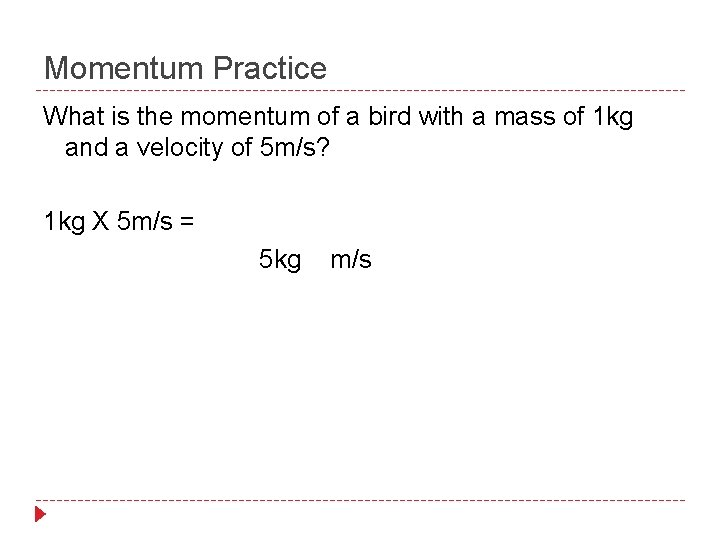Momentum Practice What is the momentum of a bird with a mass of 1 kg and a velocity of 5 m/s? 1 kg X 5 m/s = 5 kg m/s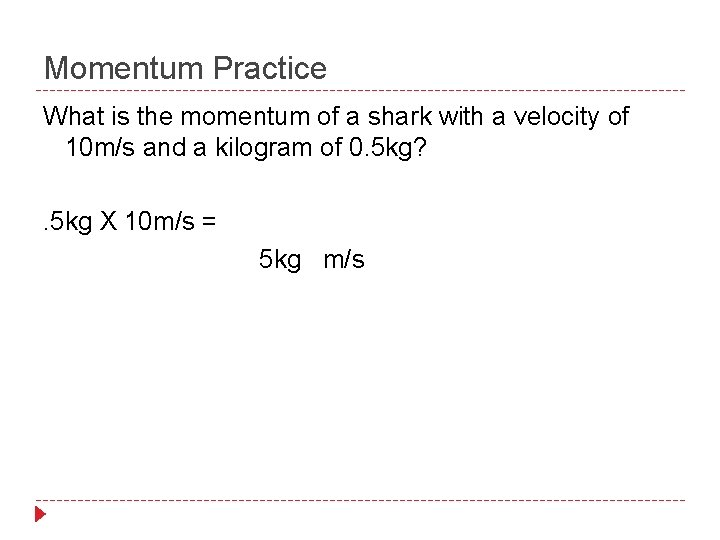Momentum Practice What is the momentum of a shark with a velocity of 10 m/s and a kilogram of 0. 5 kg? . 5 kg X 10 m/s = 5 kg m/s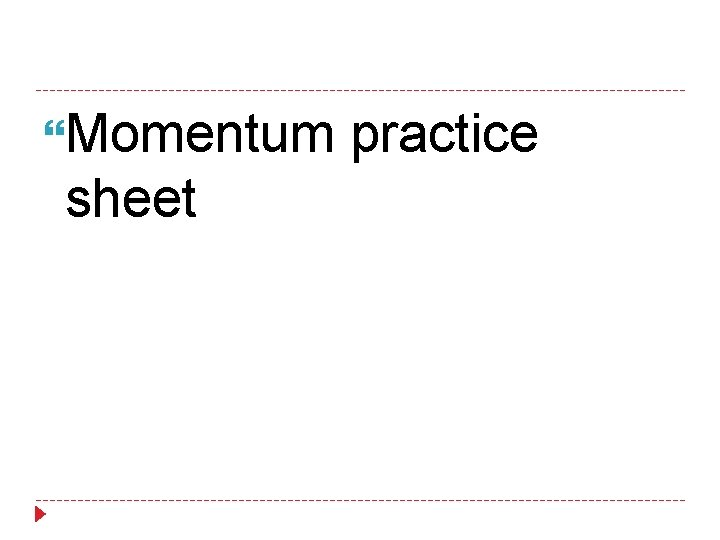Momentum practice sheetGravityWhat is Gravity? Gravity is an attractive force between two objectsThe Law of Universal Gravitation: Gravitational force depends on the masses of the two objects involved and the distance between themWhat does that mean? The closer together the objects are, the more powerful the gravitational pull The more mass an object has, the more powerful is its gravitational pullThe End!